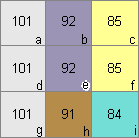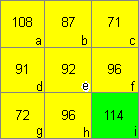# What is the algorithm used in the ASPECT function in GRID or Spatial Analyst?

Last Published: April 25, 2020

Aspect identifies the compass direction of the maximum rate of change in value from each cell to its neighbors. Aspect can be thought of as the slope direction.

Consider a 3x3 moving window over a raster, with 'a' to 'i' representing cell values. This moving 3x3 window visits each cell in the input raster and an aspect value is calculated for the cell in the center of the window, using an algorithm that incorporates the values of the cell’s eight neighbors.

`Note:If any neighborhood cells are NoData, they are assigned the value of the center cell.  An example of this is when the 3x3 window is on an edge of a raster.Taking the illustration above, the rate of change in the x direction for cell ‘e’ is calculated with the following algorithm:(dz/dx) = ((c + 2f + i) - (a + 2d + g)) / 8For example: (dz/dx) = ((85 + 170 + 84)) - (101 + 202 + 101)) / 8(dz/dx) = -8.125	 	The rate of change in the y direction for cell ‘e’ is calculated with the following algorithm:(dz/dy) = ((g + 2h + i) - (a + 2b + c))  / 8For example:(dz/dy) = ((101 + 182 + 84) - (101 + 184 + 85)) / 8(dz/dy) = -0.375Taking the rate of change in the x and y direction for cell ‘e’, aspect is calculated using:aspect = 57.29578 * atan2 (dz/dy, -dz/dx)For example:aspect = 57.29578 * atan2 (-0.375, 8.125)aspect = -2.64The above aspect value is then converted to values ranging in the compass directions (0 - 360 degrees) according to the following rule:if aspect < 0  cell = 90.0 - aspectelse if aspect > 90.0  cell = 360.0 - aspect + 90.0else  cell = 90.0 – aspectFor example:Aspect = -2.64aspect < 0Cell = 90.0 - aspectCell = 90 – (-2.64)Cell = 90 + 2.64Cell = 92.64The aspect value of 92 for cell ‘e’ = East.ReferenceBurrough, P. A. and McDonell, R.A., 1998. Principles of Geographical Information Systems (Oxford University Press, New York), p. 190.`

Article ID:000004833

Software:
• ArcMap 8 x
• ArcMap 9 x
• Legacy Products

Get help from ArcGIS experts

Contact technical support